#### The Data Science Lab### Introduction to Keras with TensorFlow

Our data science doctor provides a hands-on neural networking tutorial to explain how to get started with the popular Keras library, a high-level wrapper over TensorFlow.### Clustering Non-Numeric Data Using Python

The data science doctor explains everything you need to know about clustering data, the process of grouping items so those in a group (cluster) are similar and items in different groups are dissimilar.### Data Clustering with K-Means Using Python

Our Data Science Lab guru explains how to implement the k-means technique for data clustering, or cluster analysis, which is the process of grouping data items so that similar items belong to the same group/cluster.### Neural Network Dropout Using Python

Go hands-on with data scientist Dr. James McCaffrey as he explains neural network dropout, a technique that can be used during training to reduce the likelihood of model overfitting.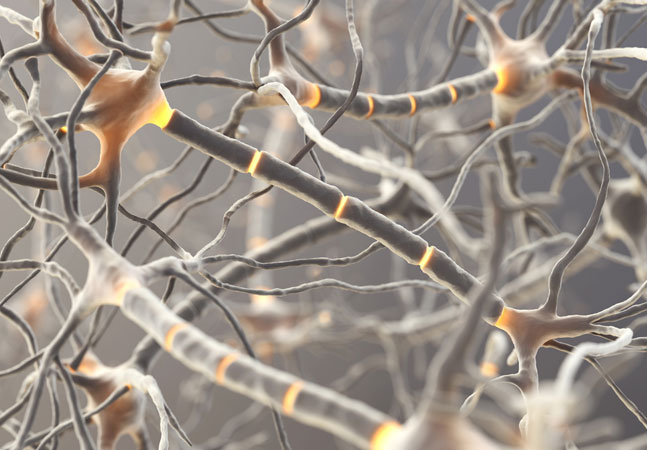### Neural Network Time Series Regression Using Python

Learn how to do time series regression using a neural network, with "rolling window" data, coded from scratch, using Python.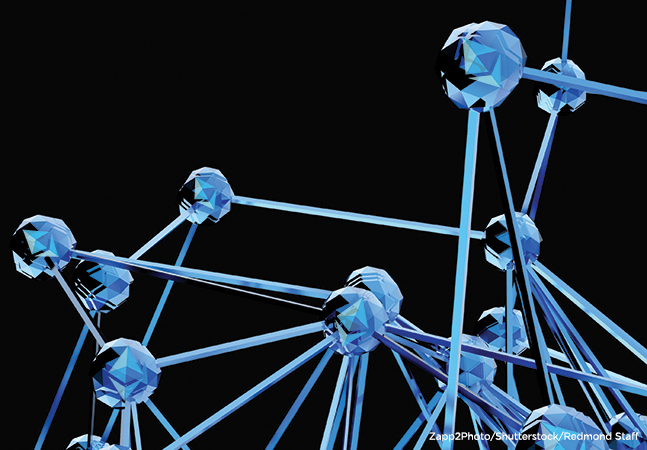### Logistic Regression Using Python

The data doctor continues his exploration of Python-based machine learning techniques, explaining binary classification using logistic regression, which he likes for its simplicity.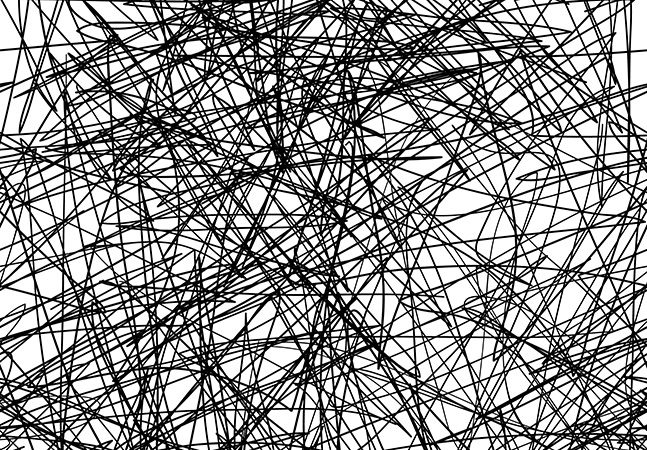### Neural Network L1 Regularization Using Python

The data science doctor continues his exploration of techniques used to reduce the likelihood of model overfitting, caused by training a neural network for too many iterations.### Neural Network Batch Training Using Python

Our resident data scientist explains how to train neural networks with two popular variations of the back-propagation technique: batch and online.### Neural Network L2 Regularization Using Python

Our data science expert continues his exploration of neural network programming, explaining how regularization addresses the problem of model overfitting, caused by network overtraining.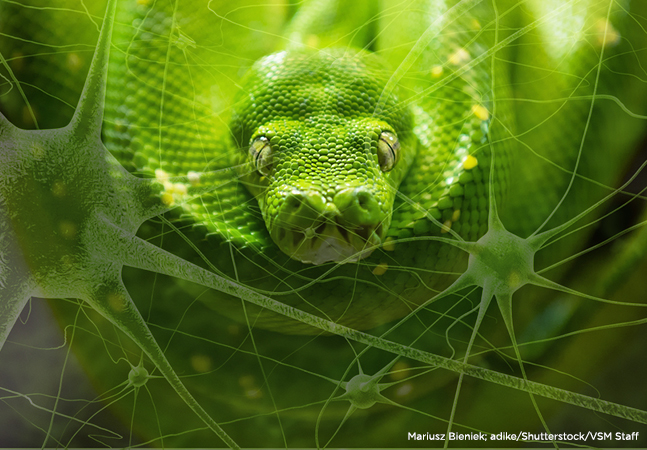### Neural Network Momentum Using Python

With the help of Python and the NumPy add-on package, I'll explain how to implement back-propagation training using momentum.### Neural Network Cross Entropy Using Python

James McCaffrey uses cross entropy error via Python to train a neural network model for predicting a species of iris flower.### Neural Network Back-Propagation Using Python

You don't have to resort to writing C++ to work with popular machine learning libraries such as Microsoft's CNTK and Google's TensorFlow. Instead, we'll use some Python and NumPy to tackle the task of training neural networks.### Neural Networks Using Python and NumPy

With Python and NumPy getting lots of exposure lately, I'll show how to use those tools to build a simple feed-forward neural network.### This R/S4 Demo Might Take You Out of Your Comfort Zone

Let's explore factor analysis again, this time using the R ability to tap into OOP, but we won't use the RC model.### Revealing Secrets with R and Factor Analysis

Let's use this classical statistics technique -- and some R, of course -- to get to some of the latent variables hiding in your data.### Data Clustering Using R

Find the patterns in your data sets using these Clustering.R script tricks.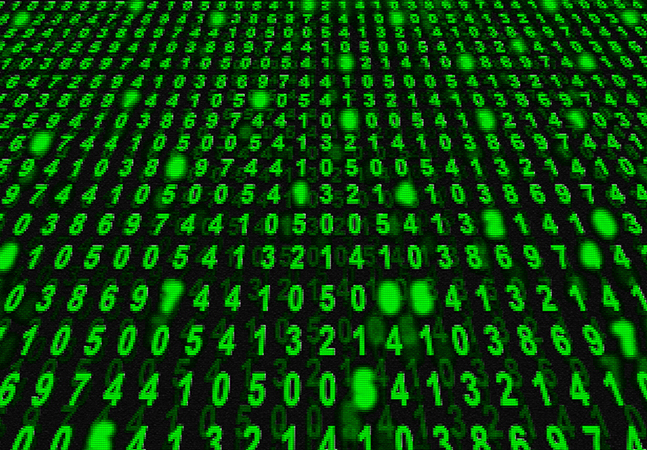### R Language OOP Using S3

The S3 OOP model is still widely used, so let's use write S3-style OOP code via the R language.### Logistic Regression Using R

I predict you'll find this logistic regression example with R to be helpful for gleaning useful information from common binary classification problems.### Neural Networks Using the R nnet Package

The R language simplifies the creation of neural network classifiers with an add-on that lays all the groundwork.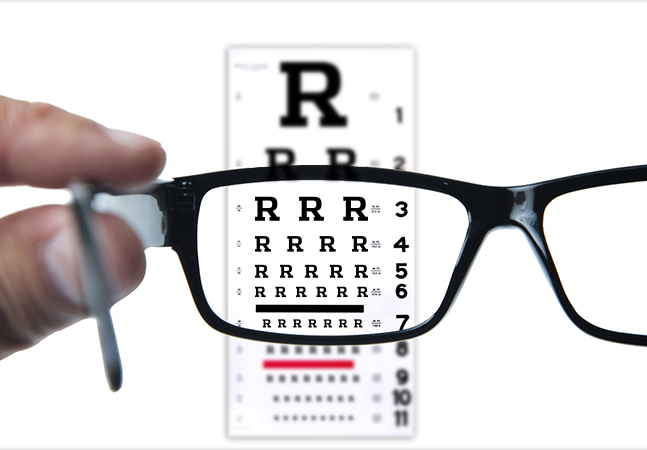### Results Are in -- the Sign Test Using R

The R language can be used to perform a sign test, which is handy for comparing "before and after" data.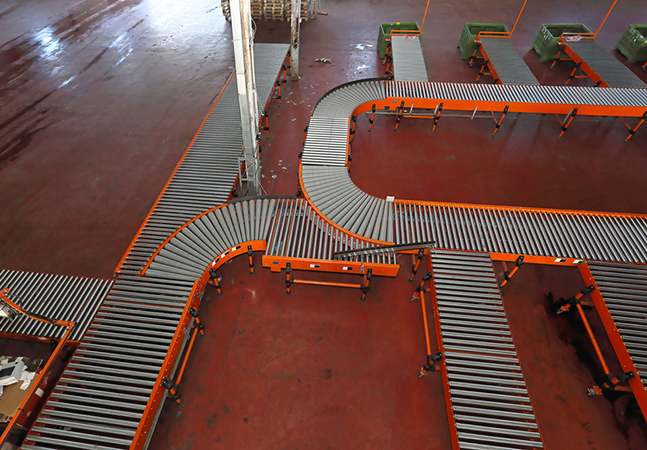### R Language Searching and Sorting

A language that's data-intensive naturally should have a way to dig into the data effectively. Here's a look at some of the R functions for searching and sorting through it all.### R Language Basic Data Structures

Vectors, lists, arrays, matrices and data frames -- a look at five of the most fundamental data structures built into R.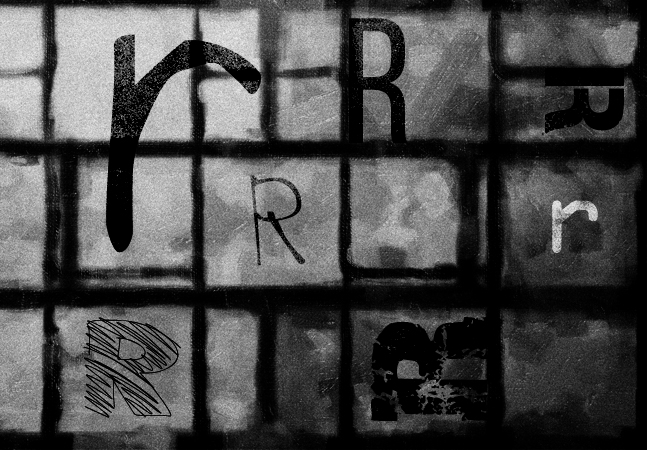### Permutations Using R

R has limited support for mathematical permutations, but it's there. Here's what R is capable of accomplishing.### How the R Language Does OOP

It's not quite like C# or Python, but the R language's object-oriented programming capabilities are getting better with each iteration. Let's take a look at what .NET developers are able do now with OOP in R6.### Classic Stats, Or What ANOVA with R Is All About

New to this type of analysis? It's a classic statistics technique that is still useful. Here's a technique for doing a one-way ANOVA using R.